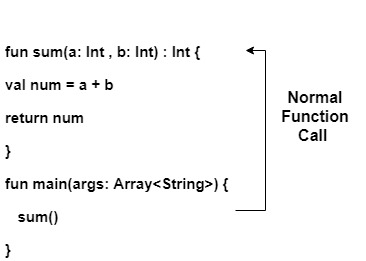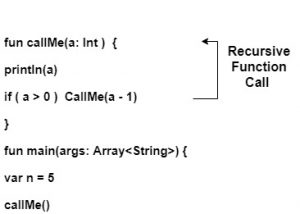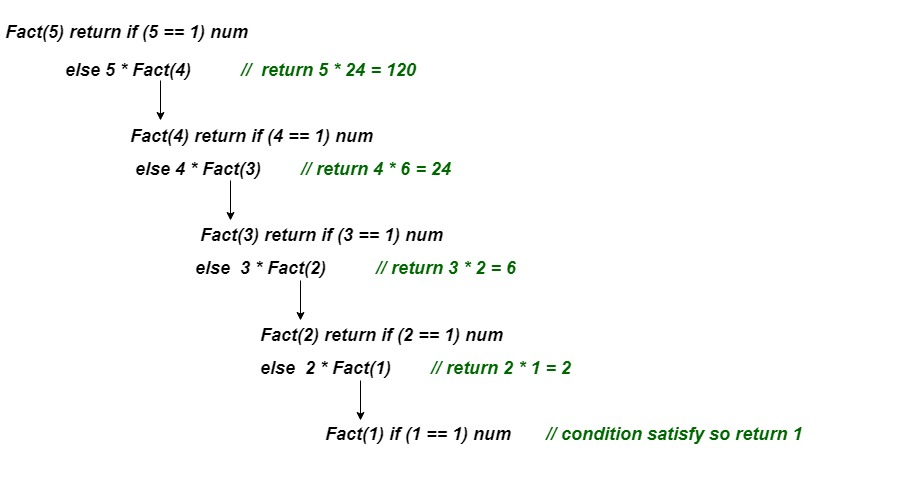GeeksforGeeks App
Open AppBrowser
Continue

## Related Articles

In this tutorial, we will learn Kotlin Recursive function. Like other programming languages, we can use recursion in Kotlin. A function that calls itself is called a recursive function and this process of repetition is called recursion. Whenever a function is called then there are two possibilities:

1. Normal function call
2. Recursive function call

## Normal function call

When a function is called from main() block then it is called a normal function call. In below example, sum() is called at a time and it executes its instruction and terminate with returning the sum of number. If we want to execute the function again then we should call sum() from the main block one more time.

Calling sum() function from main() block –## Recursive Function Call

When a function calls itself then it is called recursive function call. Every recursive function should have terminate condition else program executions enters in infinite loop and results into stack overflow error.

Calling callMe() function from its own block –Here, we have used the terminate condition if( a > 0) else it enters the infinite loop. And it prints the value from 5 down to 0.

Example 1: Find the factorial of a number without using the terminate condition.

## Java

 `// Kotlin program of factorial using recursion``fun Fact(num: Int):Long{``    ``return` `num*Fact(num-``1``) ``// no terminate condition``}   ``//main method``fun main()``{``    ``println(``"Factorial of 5 is: "` `+ Fact(``5``))``    ``// Recursive call``}`

Output:

`Exception in thread "main" java.lang.StackOverflowError`

Example 2: Find the factorial of a number with using terminate condition.

## Java

 `// Kotlin program of factorial using recursion``fun Fact(num``         ``: Int)``    ``: Long``{``    ``return` `if` `(num == ``1``) num.toLong() ``// terminate condition``        ``else` `num``        ``* Fact(num - ``1``)``}``// main method``fun main()``{``    ``println(``"Factorial of 5 is: "` `+ Fact(``5``))``    ``// Recursive call``}`

Output:

`Factorial of 5 is: 120`

### Working of factorial program –

The recursive call of Fact() is explained step by step in the following figure:-Example 3: Find the sum of elements of an array using recursion

## Java

 `// two parameters passed an array and size of array``fun sum(args``        ``: Array, index``        ``: Int)``    ``: Int``{``    ``return` `if` `(index <= ``0``) ``0` `else``(``        ``sum(args, index - ``1``)``        ``+ args[index - ``1``]) ``// recursive function call``}` `fun main()``{``    ``// array initialization``    ``val array = arrayOf(``1``, ``2``, ``3``, ``4``, ``5``, ``6``, ``7``, ``8``, ``9``, ``10``)``        ``// size of array``        ``val n``        ``= array.size val result``        ``= sum(array, n) ``// normal function call``        ``println(``"The sum of array elements is: \$result"``)``}`

Output:

`The sum of array elements is: 55`

Explanation: Here, we have initialized an array and passed as an argument to the sum() function. In each recursive call the index value decrement by one. If the index equal to zero or less than then terminate it and return the sum of all the elements.

### Advantages of recursion in Kotlin:

1. Improved code readability and maintainability: Recursive functions often make the code more concise and easier to understand, especially for complex problems.
2. Easy implementation of algorithms: Many algorithms, such as the Tower of Hanoi, can be easily implemented using recursion.
3. Reusability: Recursive functions can often be reused for similar problems, reducing the need to write new code.

### Disadvantages of recursion in Kotlin:

1. Increased memory usage: Each recursive call adds a new function call to the call stack, which can lead to stack overflow errors for large data sets.
2. Performance: Recursive algorithms are often slower than iterative algorithms, as they require more memory and CPU cycles.

My Personal Notes arrow_drop_up